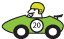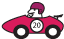### Connecting fractions, decimals and percentages

If we go back to the example of the cars passing on the highway, this table helps to show the relationship between fractions, decimals and percentages.

Cars Fraction Decimal PercentageGreen $$\dfrac{50}{100}$$ 0.5  50%Red $$\dfrac{16}{100}$$ 0.16 16%

For a percentage involving a decimal number such as 84.5%, we would write this as the fraction $$\dfrac{84.5}{100}$$ and then multiply the numerator and the denominator by 10 to get $$\dfrac{845}{1000}$$. This converts to the decimal 0.845.